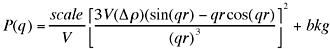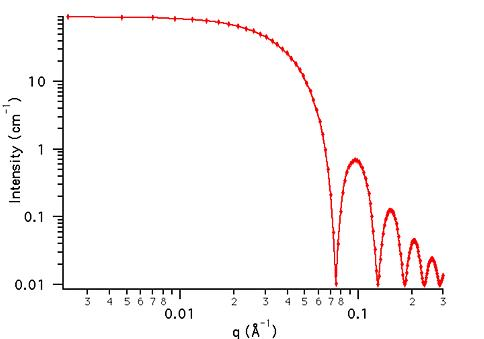MODEL

Sphere

AUTHOR/MODIFICATION

Steve Kline 06 NOV 1998

Alan Munter 08 JULY 1999, converted to Java

APPROVED FOR DISTRIBUTION

DESCRIPTION

Calculates the form factor, P(q), for a monodisperse spherical particle with uniform scattering length density. The form factor is normalized by the particle volume as described below.

Resolution smeared version is also provided.

VARIABLES

Input Variables (default values):

Parameter Variable Value
0Scale1.0
2Contrast (Å-2)1.0e-6
3Incoherent Background (cm-1)0.0

USAGE NOTES

The function calculated is:The returned value is scaled to units of [cm-1]

Scattering contrast (Dr) = SLD (core) - SLD (solvent)

Parameter (scale) and Parameter (contrast) are both multiplicative factors in the model and are perfectly correlated. One or both of these parameters must be held fixed during model fitting.

If the scale factor Parameter is set equal to the particle volume fraction, phi, then the returned value is the scattered intensity per unit volume, I(q) = phi*P(q). However, no interparticle interference effects are included in this calculation.

REFERENCE

Guinier, A. and G. Fournet, "Small-Angle Scattering of X-Rays", John Wiley and Sons, New York, (1955).

TEST DATASET

This example dataset is produced by calculating the Sphere using 128 data points, qmin = 0.001 Å-1, qmax = 0.3 (Å-1) and the above default parameter values.